# Finding the discriminative power of features to analyse how different parameters affect the rating of a book

Authors: Vrinda Narayan, Ayush Goel, Osheen Sachdev

# Motivation

Books have been a source of knowledge and information for thousands of years. With interest in books declining over the years, there is a need to engage the current audience and reignite their passion for reading. There are a wide variety of features which come into play when determining the rating of a book, including but not limited to the popularity of the author, number of pages in the book, the cover page, the summary etc.

# Literature Review

Analyzing Social Book Reading Behavior on Goodreads and how it predicts Amazon Best Sellers 
This paper takes into account reading behaviours along with other baseline features like rating, reviews etc to analyse if a book will become an Amazon Bestseller within a month of being published. To obtain reading behaviours, it extracts features such as average time to read the book, number of status posts, etc from status posts posted by users. They tried out various machine learning models but obtained the best results using logistic regression (with an accuracy of 88.72\%).

Success in books: predicting book sales before publication 
This paper takes into account the features from the author(Popularity), book(Genre, publishing month) and publisher to predict the sales of the book. Popularity of the author is estimated by the number of views on the wikipedia page of the author and the sales of previous books as reported on the bookscan website. They employed a new machine learning model “Learning to place” which outperformed the other learning methods for this task.

# Curating the dataset

We created the dataset by scraping information regarding books from Goodreads using Python beautifulsoup. We scraped random books, uniquely identified by their ISBN Number. The collected dataset consists of 20274 books with features given below.

1. ISBN (Alphanumeric): ISBN number of book.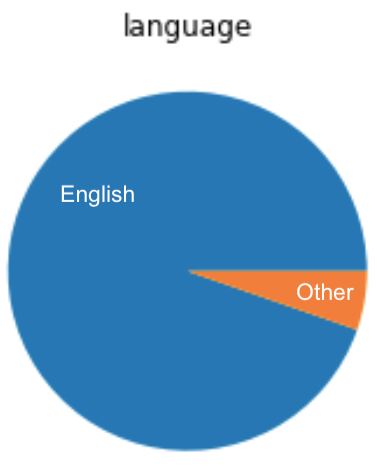Figure 1: Data distribution of(a) numerical data (b) genres and (c) language

# Preprocessing

We one hot encoded the genres and removed outliers from the training data. The preprocessing steps that were followed are shown in Figure 2.

We separated 20% of the data as Testing Data and the test set had size 4052 and final train data had size 16206.

# Feature Selection

Feature selection was performed on linear regression.
Since our problem was to predict the rating before a book is published, considering features like Rating Count, Review Count and Number of Awards defeats our purpose since these values are known after a book is published. Hence these features were removed.
We also removed the book format from our set of features since the same book can be released in multiple formats and will have no influence in our ratings. We took log of Author Followers since the feature consisted of really large values with a large range. All these changes were further corroborated by the weights of our linear regression model.

# Methodology and Results

Evaluation Metric
While performing EDA on our dataset, we realized the majority of the ratings were concentrated around 3.7 to 4.3 with mean being at 3.97 as seen from Figure 1. To accurately analyze the performance of our model, there was a need to penalize incorrectly predicted values further from the mean more as compared to values closer to the mean. Paper  proposed a new evaluation metric SERA which provides valid and useful insights into the performance of models in imbalanced regression tasks. We used a similar metric with the formula given below.

Predict rating from statistical features

We approached our problem as both classification as well as a regression problem to understand the difference between the two and analyze what is better suited for our case.

We tried regression on book rating using linear regression (Figure 3), decision tree regressor, support vector regressor, artificial neural networks, etc. The results can be seen in Table 1.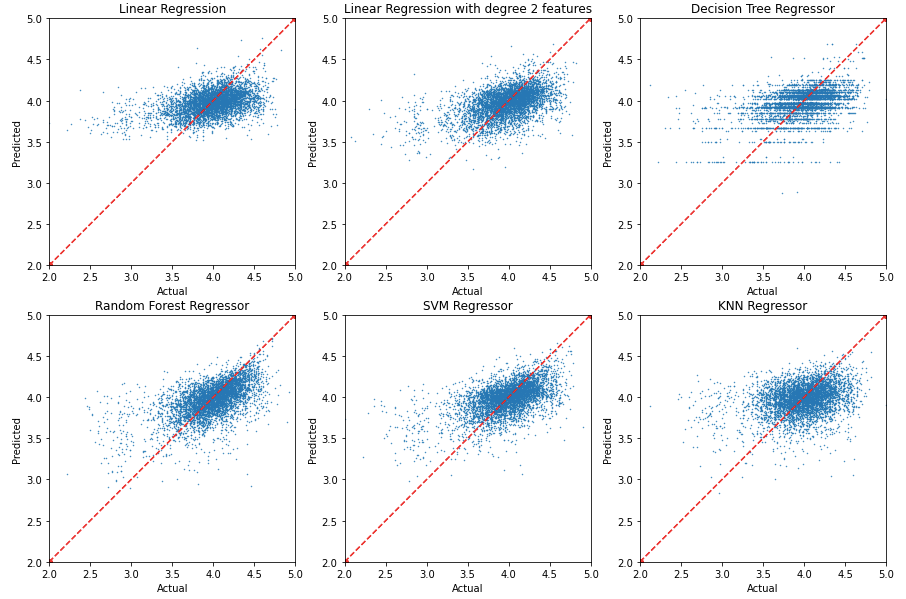Figure 4: Scatterplot of actual rating vs predicted rating for regression models

The scatterplot in Figure 4 shows the performance of the models in predicting the ratings. For models with more scattered plots, the variance is high and for plots that are more horizontal, bias is high.

Since most of our models were underfitting, we also tried some ensemble techniques like AdaBoost, Bagging, Randomforest and XGBoost to improve the performance of decision trees and K nearest neighbours.

For classification, we divided the Rating which was a linear variable into buckets of different sizes like 0.5, 0.2, 0.05, 0.01 and 0.005 and performed classic Classification Algorithms. The evaluation metric used was however MSE since our original task was a regression task and converting to a classification task is part of our methodology and not the problem statement.

Predict rating from Book Cover

We first converted the book images to NumPy arrays using the PIL library and did padding to make all the images of the same size (102 X 50 X 3). We normalized the data and converted this to a single array (feature size = 15300) and took it as our input feature set for ANN. We tried different activation functions, layer size and number of layers for our ANN model and our final ANN model had 8 layers with size 10, 30, 50, 100, 40, 30, 20, 10.

We also tried CNN with the normalized image as input with 5 hidden layers. The structure can be seen in Figure 5.

Figure 6 shows the performance of ANN on book covers in predicting the rating of books. ANN on Book Covers gave a training MSE of 0.1174 and Validation MSE of 0.2500.

Predict rating from Summary

To predict the rating from the summary of the book, we first tokenized the summary and performed POS tagging on the words. We only considered Nouns, Verbs and Adjectives which had a frequency ≥ 10 in our corpus. Words like life, world, new were some of the most common words present in summaries. The total number of words considered were 11,214.

Each summary was one-hot-encoded on these words, giving 11214 features for each summary.

We performed linear regression (on top 2000 words), neural networks without regularization and neural networks with regularization (L2, alpha = 0.0001). For both the neural networks, we had 3 hidden layers with size 50 and activation functions tanh, relu and relu respectively as can be seen from Figure 7.

Doc2Vec is an unsupervised algorithm to generate sentence embeddings (score). We also used doc2vec from gensim, trained it on our data and generated the embeddings of the summary. It returned a feature vector of size 100 which was inputted into a Random Forest Model as input.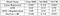Table 4: performance of models in predicting the rating of a book from its summary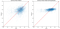Figure 8: Scatterplot for actual vs predicted ratings using summary from (a) ANN (b) ANN with regularisation

The performance of models trained on summary can be seen in Table 4.

Figure 8 shows a comparison of the performance of ANN with and without regularisation.

# Discussion

Rating from numerical data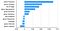Figure 9: Weights of features in linear regression for predicting rating from numerical data

As seen from Figure 9, features like Author Followers, Number of Pages play a significant role in determining the rating of a book. An interesting observation to note was that Children’s, Sequential Art and Romance books are more likely to be rated higher whereas Literature and Historical books are less likely to be rated higher.

Rating from Book Cover

From the performance of models on book covers in predicting rating, we concluded that there is not a direct correlation between the Book Covers and rating of a book as other models were performing much better.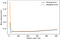Figure 10: Change in MSE on increasing the number of hidden units in the ANN for predicting rating from summary

We tried hidden layer sizes [8, 16, 32, 64, 128, 256, 512]. It can be observed from Figure 10 that increasing hidden layer size was not causing the model to overfit. The error became constant after hidden layer size > 32. Thus, Book Covers were not contributing to our model, just adding noise and we decided to not include it in our final ensemble model.

Rating from Summary

The words with highest value of weights in linear regression on rating from summary can be seen in Figure 11.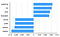Figure 11: Weights of some words in linear regression on Summary for predicting the rating of a book

We can see words like preparing, foe, exile etc are buzzwords which are more likely to capture the users attention and hence play a more important role in determining the engagement of the user.

# Final Model

We split the data into 80–20 train validation split and numerical and summary models. We fed the predicted output of these models to a Linear Regression Model as input to get the final model. The detailed architecture can be seen in Figure 12.

There is a slight improvement in the performance of the Final Model as compared to the two input models as can be seen in Table 5.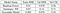Table 5: Performance of the final model as compared to the individual models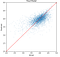Figure 11: Scatterplot of actual vs predicted rating for the final model on testing data

On analysis of the final layer coefficients, the weight assigned to Summary NN was 0.2286 and that of Random Forest was 1.0092. Thus we can see that numerical data plays a much significant role in determining the rating of a book as compared to the summary.

# Conclusion

Through the medium of this project, we tried various Machine Learning and Neural Network models which helped us in clearing our understanding of ML Concepts. We faced various challenges while approaching our problem and were not getting great results initially. This motivated us to try new techniques and led us to discover new concepts related to Machine Learning like Bagging, Ada Boost, Regularization etc. We discovered how different models perform better depending on the type of data and tackled various challenges like over-fitting and under-fitting. We also learned about how creating a custom metric is better suited for certain kinds of problems.

Key Findings:

1. The influence of author followers is the highest as analysed by the weights of the linear regression model.

We also defined a custom metric suitable to analyze the performance of imbalanced regression tasks. Our final model was able to achieve Testing MSE as low as 0.072 and a score of 0.358 on our custom metric.

# References

 Suman Maity, Abhishek Panigrahi, and Animesh Mukherjee. Analyzing social book reading behavior on Goodreads and how it predicts amazon best sellers, 09 2018.

 Nuno Moniz Rita P. Ribeiro. Imbalanced regression and extreme value prediction, 2020.

 Xindi Wang, Burcu Yucesoy, Onur Varol, Tina Eliassi-Rad, and Albert-L ́aszl ́o Barab ́asi. Success in books: predicting book sales before publication, 2019.

# Acknowledgements

A special Thanks to the professor Dr. Jainendra Shukla and the Teaching Assistants!

Monsoon2020 course: Machine Learning

Instructor: Dr. Jainendra Shukla

Project TA: Anmol Singhal